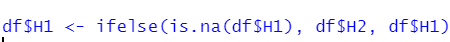Question: Replacing NA values of a column using values from another column
0
SGMS70 wrote:

Hi guys,

I am trying to manipulate my dataset but I have two conditions and I haven't dealt with such an issue before.

For example I have:

`````` ID  T   value(%)   H1   sex     H2

1   T1    38.1     15.2    F    14.5

1   T2    39.5     14.2    M    14.5

10  T1    34.1      N      F    11.4
``````

I want to replace the NA value of H1 column, with the value in the corresponding H2's row (11.4). I have previously merged datasets based on the ID so H1 and H2 are sorted based on ID. When I try to replace the NA value of H1 with the values in H2, it says "Error in `\$<-.data.frame`(`*tmp*`, H1, value = c(14.5, 14.1, : replacement has 298 rows, data has 609".

So I guess my problem is that I want to replace my NA values using another column's rows. Is there a way of doing this?

Any help would be greatly appreciated.

R data manipulation • 94 views
modified 12 days ago by genomax91k • written 13 days ago by SGMS70
1
Sam3.2k wrote:

If you have data.table installed, and assuming your data is stored in `dat`

``````library(data.table)
dat <- as.data.table(dat)
dat[is.na(H1), H1:=H2]
``````

should do

0
Hamid Ghaedi570 wrote:

Say your data frame is `df` using base R you can do:

``````df[is.na(df\$H1), ]\$H1 <- df[is.na(df\$H1), ]\$H2
``````

Or using `ifelse`: